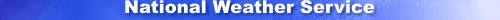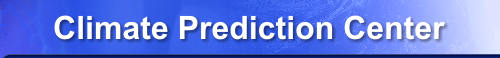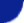Site Map News Organization SearchHOME > Monitoring_and_Data > Oceanic and Atmospheric Data > Reanalysis: Atmospheric Data > wgrib2-reduced_gaussian_grid

Introduction

Regular Gaussian grids are not space efficient because the number of grid points on a Gaussian latitude does not vary with latitude. For example, you don't need as many points near the poles as next to the equator to describe the same spatial scales. With a reduced Gaussian grid, the number of grid points on a Gaussian latitude varys from large near the equator to small near the poles. This reduces the number of grid points, reduces calculations and speeds up the model. One draw back for the users are they often have convert the grid to a regular Gaussian grid for plotting, interpolation to another grid or making a regional subset.

The -reduced_gaussian_grid option converts from a reduced Gaussian grid to a regular Gaussian grid. This option has two interpolation options. The "linear" interpolation means that the grid points are interpolated linearly from the surrounding two points on the Gaussian latitude. "Neighbor" interpolation will use the value from the nearest grid point on the Gaussian latutude.

Interpolation

The -reduced_gaussian_grid option interpolates using points on the same Gaussian latitude. There are two basic interpolation techniques, linear and neighbor.
1. linear interpolates linearly using the two surrounding grid points on the same Gaussian latitude assuming that both grid points have valid values.
2. neighbor interpolation will take the value of the nearest grid point on the same Gaussian latitude assuming that both grid points have valid values.

Some fields have undefined values. Therefore, in some cases you may have to extrapolate a value.

D------x-------U         (Gaussian latitude)
D=defined value, U=undefined values
x=location of value that is desired

Option 1: no extrapolation, value at x is undefined
Option 2: extrapolation, value at x is the value D
Option 3: x has the value D if x is closer to "D" than "U"
otherwise it has a undefined values

Option 1: default, Option 2: extrapolate, Option 3: not supported

The supported interpolation types are

1. linear : linear, no extrapolation
2. linear-extrolate : linear, extrapolation
3. neighbor : nearest neighbor, no extrapolation
4. neighbor-extrapolate : nearest neighbor, extrapolation

Example

See that reduced_gaussian_surface_jpeg.grib2 is a reduced Gaussian grid
bash-4.1\$ wgrib2 reduced_gaussian_surface_jpeg.grib2 -grid
1:0:grid_template=40:winds(N/S):
thinned global Gaussian grid: (-1 x 64) units 1e-06 input WE:NS output raw
number of latitudes between pole-equator=32 #points=6114
lat 87.864000 to -87.864000
lon 0.000000 to 357.188000 by -2147.483647
#grid points by latitude: 20 27 36 40 45 50 60 64 72 75 80 90 90
96 100 108 108 120 120 120 128 128 128 128 128 128 128 128 128 128 128 128 128
128 128 128 128 128 128 128 128 128 128 128 120 120 120 108 108 100 96 90 90
80 75 72 64 60 50 45 40 36 27 20

Write regular Gaussian grid in new.grb
bash-4.1\$ wgrib2 reduced_gaussian_surface_jpeg.grib2 -reduced_gaussian_grid new.grb -1 linear
1:0:d=2007032312:TMP:surface:anl:

Check that new.grb is a regular Gaussian grid
bash-4.1\$ wgrib2 new.grb -grid
1:0:grid_template=40:winds(N/S):
Gaussian grid: (128 x 64) units 1e-06 input WE:NS output WE:SN
number of latitudes between pole-equator=32 #points=8192
lat 87.864000 to -87.864000
lon 0.000000 to 357.187500 by 2.812500

Usage

-reduced_gaussian_grid OUT -1 INTERPOLATION
OUT = output file
grib2, regular Gaussian grid
The interpolation is linear on the Gaussian latitude
but will be made selectable in the future
-1 = means interpolate to a regular Gaussian grid
In the future, this parameter will extened
for convertion to a reduced Gaussian grid.
INTERPOLATION = linear, neighbor, linear-extrapolate, neighbor-extrapolate